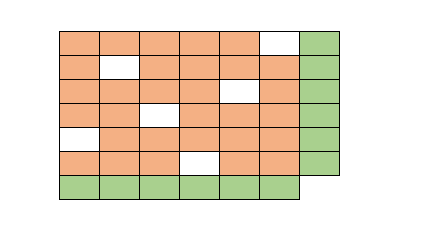## Tuesday, August 29, 2023

### Matrix balancing: introduction

Matrix Balancing Models are often used in economic modeling exercises: they create consistent data sets from data originating from different, conflicting data sources. A standard example is updating a matrix subject to given row and column sums. An example can look like:Update orange cells subject to row/column sums

The empty cells are zero, and they should remain zero. In other words, we need to preserve sparsity. Often, we have non-negativity restrictions on the values. The mathematical model can look like this:

Matrix Balancing Problem
\begin{align}\min_{\color{darkred}A}\>&{\bf{dist}}(\color{darkred}A,\color{darkblue}A^0)\\ & \sum_i \color{darkred}A_{i,j} = \color{darkblue}c_j && \forall j\\ & \sum_j \color{darkred}A_{i,j} = \color{darkblue}r_i && \forall i \\&\color{darkred}A_{i,j}=0 &&\forall i,j|\color{darkblue}A^0_{i,j}=0\\ &\color{darkred}A_{i,j}\ge 0 \end{align}

In this model, we have high confidence in the data for $$\color{darkblue}c_j$$ and $$\color{darkblue}r_i$$, but less confidence in $$\color{darkblue}A^0_{i,j}$$. The exogenous values $$\color{darkblue}A^0$$ are sometimes called priors. Some popular functional forms for the objective are:

• Cross-entropy:
$$\displaystyle{\min \sum_{i,j} \color{darkred}A_{i,j} \ln \frac{\color{darkred}A_{i,j}}{\color{darkblue}A^0_{i,j}}}$$,
$$\displaystyle{\min \sum_{i,j} \left(\color{darkred}A_{i,j}-\color{darkblue}A^0_{i,j}\right)^2}$$,
$$\displaystyle{\min \sum_{i,j} \left(\frac{\color{darkred}A_{i,j}}{\color{darkblue}A^0_{i,j}}-1\right)^2}$$

### Spatial data

In our application, we deal with spatial data. Basically, I am talking about acres planted for a given crop (a bit simplified; we make further distinctions such as irrigation regime). The regions we are talking about are HUCs: Hydrologic Unit Codes. This is a regional structure from USGS (United States Geological Survey). We have 6-digit, 4-digit and 2-digit levels. Huc-6 regions are the most disaggregated.

Huc-2 codes can be just 1 digit if the leading 0 is dropped. This depends a bit on the representation: as string or as integer. Integers lose leading zeros. These little details can cause problems when dealing with actual data sets. E.g. the huc-2 codes can look like:

or

It is good to define once and for all how they should look like.

The huc-6 regions are embedded in a huc-4 region, which is again part of a huc-2 region. This forms a hierarchical structure. At the top, we have national data. Often, the national data is considered the bible: we trust those numbers. One reason is that those national numbers are well-known: agricultural economists are very familiar with these statistics.

A beautiful, old-fashioned, retro-style map  is:

A more modern, d3-based   interactive choropleth map can look like:

Here is a small example of huc 2, huc 4, and huc 6 codes:

### Multi-level matrix balancing

The data points (or priors) for each region $$\color{darkblue}a^0_r$$ are imprecise. We have a margin of error $$\color{darkblue}E_r$$ for each of them. We can use a multi-level matrix balancing model to create a consistent data set. Such a model can look like:

Multi-level Matrix Balancing Problem
\begin{align}\min\>&\sum_{r \in h2\cup h4\cup h6}\color{darkblue}w_r\left(\color{darkred}a_r-\color{darkblue}a^0_r\right)^2\\ & \color{darkred}a_{h4} = \sum_{h6 \in h4} \color{darkred}a_{h6} && \forall h4\\ &\color{darkred}a_{h2} = \sum_{h4 \in h2} \color{darkred}a_{h4} && \forall h2 \\& \color{darkblue}a^0_{\mathit{US}} = \sum_{h2} \color{darkred}a_{h2} \\ &\color{darkred}a_{r}=0 &&\forall r|\color{darkblue}a^0_{r}=0 \\ & \color{darkred}a_{r}\in [\color{darkblue}\ell_r,\color{darkblue}u_r] \\ &\color{darkblue}\ell_r = \max\{\color{darkblue}a^0_r - \color{darkblue}E_r, 0\} \\ & \color{darkblue}u_r = \color{darkblue}a^0_r + \color{darkblue}E_r \\ & \color{darkblue}w_r = \frac{1}{\color{darkblue}u_r-\color{darkblue}\ell_r} \end{align}

Notes:
• Identifiers colored red are endogenous (decision variables), while the blue symbols are exogenous (parameters).
• The $$r$$ index refers to all regions (huc 2, 4, and 6).
• The huc-2 data has to add up to the national level.
• Some variations are possible here, e.g. in how we specify the weights $$\color{darkblue}w_r$$ and the functional form of the objective function. In our case, we could use hard bounds based on the margin of error. If that is problematic (too tight), we can use some elastic formulation.
• The real problem is rather large (with many more data for each region). We can solve this in one big model or as a series of smaller models.
• The convex quadratic objective allows us to use a general purpose NLP solver (such as CONOPT), or a more specialized algorithm (such as the conic solver in Cplex).

The output of the model: optimal levels of $$\color{darkred}a_r$$ form a consistent data set. The huc 6 levels add up to huc 4, the huc 4 levels add up to huc 2, and the huc 2 levels add up to national data. We model this hierarchy with simultaneous equations.

### Conclusions

Some models require additional math programming models just to create consistent data sets. This is a nice example of that.

Earlier in discussions about this problem, there were ideas to implement an algorithm for this. I would not know how to do that. Instead, using a formal optimization model, is quite straightforward and rather elegant, I think.

### References

1. USGS, Hydrologic Unit Maps, https://water.usgs.gov/GIS/huc.html
2. The JavaScript library for bespoke data visualization, https://d3js.org/

### Appendix: GAMS code

 $onText 3-level matrix balancing demo$offText  *---------------------------------------------------------------* read sets from huc.inc* the readme part is shown below*---------------------------------------------------------------  *---------------------------------------------------------------* Regions extracted from c:\tmp\huc\huc6main.geojson* Contents:*   set  huc0:  superset, all huc regions*   set  huc6:  6 digit huc regions*   set  huc4:  4 digit huc regions*   set  huc2:  2 digit huc regions*   set  hucisin:  region 1 is inside region 2*   parameter hucacres:  size of region in acres** Generated: 2023-08-26*--------------------------------------------------------------- $include c:\tmp\huc\huc.inc *---------------------------------------------------------------* generate some dummy data*--------------------------------------------------------------- * shorter names for setsalias(r,huc0);alias(h6,huc6),(h4,huc4),(h2,huc2); parameter a0(r) 'prior estimates' e(r) 'margin of error' a0us 'national data'; a0(h6) = uniform(0,100);e(h6) = uniform(0,100);a0(h4) = sum(hucisin(h6,h4),a0(h6));e(h4) = uniform(0,50);a0(h2) = sum(hucisin(h4,h2),a0(h4));e(h2) = uniform(0,20);a0us = sum(h2,a0(h2)) + 100; *---------------------------------------------------------------* matrix balancing model*--------------------------------------------------------------- parameters lo(r) 'd-r' up(r) 'd+e' w(r) 'weights';lo(r) = max(a0(r)-e(r),0);up(r) = a0(r)+e(r);w(r)$(up(r)>lo(r)) = 1/(up(r)-lo(r));  variable a(r);a.lo(r) = lo(r);a.up(r) = up(r); variable z 'objective'; equations   obj      'minimize squared weighted deviations'   sum6(h4) 'h4 = sum h6'   sum4(h2) 'h2 = sum h4'   sum2     'US = sum h2'; obj..  z =e= sum(r, w(r)*sqr(a(r)-a0(r)));sum6(h4).. a(h4) =e= sum(hucisin(h6,h4),a(h6));sum4(h2).. a(h2) =e= sum(hucisin(h4,h2),a(h4));sum2.. a0us =e= sum(h2,a(h2)); model matbal /all/;solve matbal using qcp minimizing z;